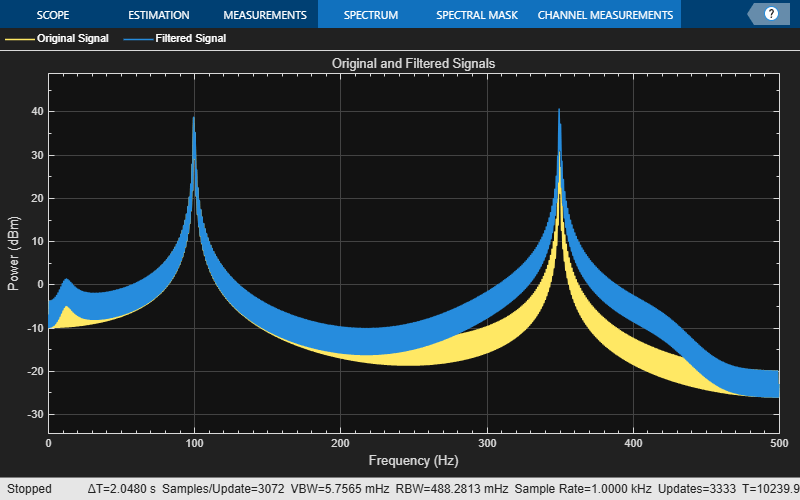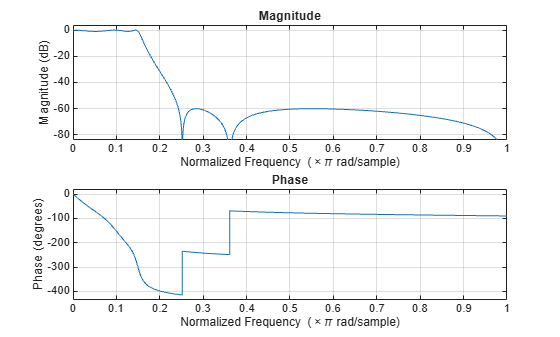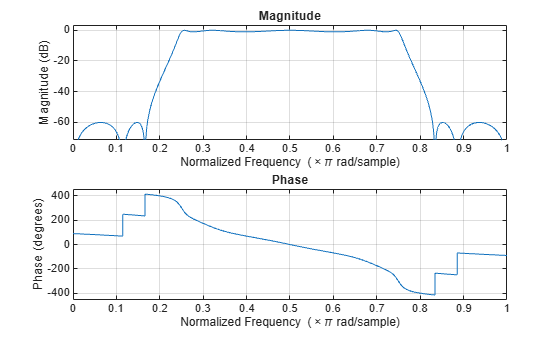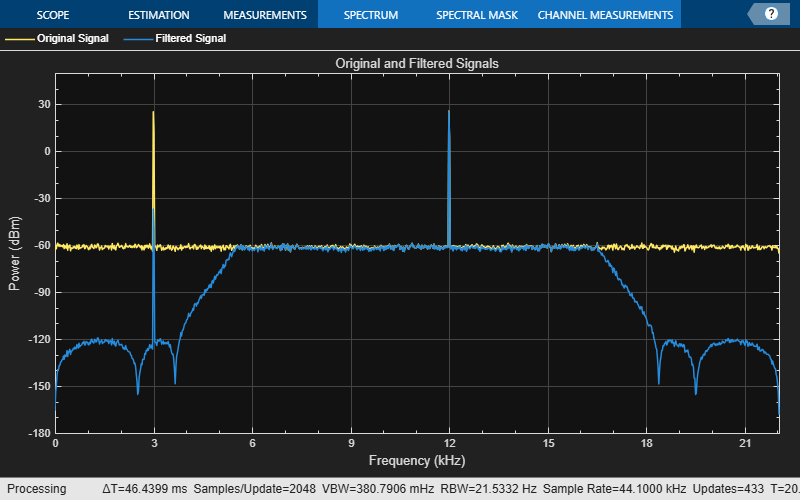# dsp.FourthOrderSectionFilter

Implement cascade of fourth-order section filter

Since R2019a

## Description

The `dsp.FourthOrderSectionFilter` implements a cascade of fourth-order section filters.

## Creation

### Syntax

``fos = dsp.FourthOrderSectionFilter``
``fos = dsp.FourthOrderSectionFilter(num,den)``
``fos = dsp.FourthOrderSectionFilter(Name,Value)``

### Description

````fos = dsp.FourthOrderSectionFilter` returns a `FourthOrderSectionFilter` object, `fos`, that implements a cascade of fourth order filter sections.```

example

````fos = dsp.FourthOrderSectionFilter(num,den)` returns a `FourthOrderSectionFilter` object with the `Numerator` property set to `num` and the `Denominator` property set to `den`.```

example

````fos = dsp.FourthOrderSectionFilter(Name,Value)` returns a `FourthOrderSectionFilter` object with each specified property name set to the specified value. You can specify additional name-value pair arguments in any order.Example: ```fos = dsp.FourthOrderSectionFilter('Numerator',num,'Denominator',den)``````

## Properties

expand all

Numerator coefficients of the filter, specified as an L-by-5 matrix, where L is the number of filter sections. The size of this property cannot change when the object is locked. However, the values can be modified.

Data Types: `single` | `double`
Complex Number Support: Yes

Denominator coefficients of the filter, specified as an L-by-5 matrix or an L-by-4 matrix, where L is the number of filter sections. The leading denominator coefficients are assumed to be `1` always. If the denominator is of size L-by-4, one(s) are appended to make the size L-by-5. If the denominator is of size L-by-5, the first column values are ignored and appended with 1s. The size of this property cannot change when the object is locked. However, the values can be modified.

Data Types: `single` | `double`
Complex Number Support: Yes

## Usage

### Syntax

``y = fos(x)``

### Description

````y = fos(x)` filters the input signal using the specified fourth-order section filter to produce the filtered output, `y`.```

### Input Arguments

expand all

Input signal, specified as a vector or a matrix.

The input can be a variable-sized signal, that is, the frame size of each channel (number of rows) can change even after the object is locked. However, the number of channels (number of columns) cannot change.

Data Types: `single` | `double`
Complex Number Support: Yes

### Output Arguments

expand all

Filtered output, returned as a vector or a matrix. The output has the same size, data type, and complexity as the input signal.

Data Types: `single` | `double`
Complex Number Support: Yes

## Object Functions

expand all

 `fvtool` Visualize frequency response of DSP filters `freqz` Frequency response of discrete-time filter System object `impz` Impulse response of discrete-time filter System object `info` Information about filter System object `coeffs` Returns the filter System object coefficients in a structure `cost` Estimate cost of implementing filter System object `grpdelay` Group delay response of discrete-time filter System object
 `step` Run System object algorithm `release` Release resources and allow changes to System object property values and input characteristics `reset` Reset internal states of System object `clone` Create duplicate System object `isLocked` Determine if System object is in use

## Examples

collapse all

Filter a noisy sinusoidal signal using the `dsp.FourthOrderSectionFilter` object. Visualize the original and filtered signals using a spectrum analyzer.

Input Signal

The input signal is the sum of two sine waves with frequencies 100 Hz and 350 Hz. Add zero-mean white Gaussian noise with a standard deviation of 1e-4 to the sum of sine waves. The sample rate is 1000 Hz.

```frameSize = 1024; fs = 1000; Sine1 = dsp.SineWave(5,100,'SamplesPerFrame',1024,'SampleRate',fs); Sine2 = dsp.SineWave(2,350,pi/2,'SamplesPerFrame',1024,... 'SampleRate',fs); x = Sine1()+Sine2()+1e-4.*randn(Sine1.SamplesPerFrame,1);```

Fourth-Order Section (FOS) Filter Coefficients

The numerator and denominator coefficients for the FOS filter are obtained using `designParamEq` which is part of Audio Toolbox:

```%N = [2,4]; %gain = [5,10]; %centerFreq = [0.025,0.75]; %bandwidth = [0.025,0.35]; %mode = 'fos'; %[num,den] = designParamEQ(N,gain,centerFreq,bandwidth,mode,'Orientation','row'); num = [1.0223 -1.9368 0.9205 0 0 1.5171 2.3980 1.4317 0.6416 0.2752]; den = [-1.9368 0.9428 0 0 2.0136 1.9224 1.0260 0.3016];```

Initialize Filter and Spectrum Analyzer

Construct the FOS IIR filter using the `num` and `den` coefficients. Construct a spectrum analyzer to visualize the original sinusoidal signal and the filtered signal.

```fos = dsp.FourthOrderSectionFilter('Numerator',num,... 'Denominator',den); scope = spectrumAnalyzer(... 'SampleRate',fs,... 'PlotAsTwoSidedSpectrum',false,... 'FrequencyScale','linear',... 'Method','welch',... 'Title','Original and Filtered Signals',... 'ShowLegend',true,... 'ChannelNames',{'Original Signal','Filtered Signal'});```

Filter the input signal, and visualize the original and filtered spectrums.

```for i = 1:10000 y = fos(x); scope([x,y]); end release(scope);```Design a lowpass fourth-order section (FOS) filter using the `fdesign` function. Using this filter, filter a noisy sinusoidal signal with two tones, one at 3 kHz, and the other at 12 kHz.

Design a fifth-order filter using the elliptic method in the `'df2tsos'` structure. Use L-infinity norm scaling in the frequency domain. Specify the passband frequency to be 0.15$\pi$ rad/sample and the stopband frequency to be 0.25$\pi$ rad/sample. Specify 1 dB of allowable passband ripple and a stopband attenuation of 60 dB.

```Fp = 0.15; Fst = 0.25; Ap = 1; Ast = 60;```

The filter coefficients are scaled using an `fdopts.sosscaling` object. The scaling object is defined to have no numerator constraints, and the `ScaleValueConstraint` is set to `'unit'`, specifying the scaling to be unity scaling.

```fdo = fdopts.sosscaling; fdo.NumeratorConstraint='none'; fdo.ScaleValueConstraint='unit'; f = fdesign.lowpass('Fp,Fst,Ap,Ast',Fp,Fst,Ap,Ast); hFilter = design(f,'ellip','SystemObject',true,... 'FilterStructure','df2tsos','SOSScaleNorm','Linf',... 'SOSScaleOpts',fdo)```
```hFilter = dsp.SOSFilter with properties: Structure: 'Direct form II transposed' CoefficientSource: 'Property' Numerator: [3x3 double] Denominator: [3x3 double] HasScaleValues: true ScaleValues: [1 1 1 1.0000] Show all properties ```

Visualize the lowpass frequency response of the designed filter using `fvtool`.

`fvtool(hFilter)`Convert the lowpass filter to a bandpass filter using the `iirlp2bp` function.

`[num,den] = iirlp2bp(hFilter.Numerator,hFilter.Denominator,Fp,[0.25,0.75])`
```num = 3×5 0.2456 0 -0.2456 0 0 0.4175 -0.0000 -0.4206 -0.0000 0.4175 0.4281 -0.0000 -0.6433 -0.0000 0.4281 ```
```den = 3×5 1.0000 -0.0000 0.5088 0 0 1.0000 -0.0000 0.0060 -0.0000 0.8657 1.0000 -0.0000 0.5160 -0.0000 0.5283 ```

Create a fourth-order section filter using these numerator and denominator coefficients.

`fos = dsp.FourthOrderSectionFilter(num,den)`
```fos = FourthOrderSectionFilter with properties: Numerator: [3x5 double] Denominator: [3x5 double] ```

Visualize the frequency response of the fourth-order section filter using `fvtool`.

`fvtool(fos);`Filter a noisy input signal with the fourth-order section filter. Visualize the spectra of the original signal and the filtered signal using the spectrum analyzer.

The input is a sum of two sine waves with frequencies 3 kHz and 12 kHz, respectively. The input sample rate is 44.1 kHz, and the frame size is set to 1024 samples.

```fs = 44100; FrameLength = 1024; SINE1 = dsp.SineWave('SamplesPerFrame',FrameLength,'SampleRate',fs,'Frequency',3000); SINE2 = dsp.SineWave('SamplesPerFrame',FrameLength,'SampleRate',fs,'Frequency',12000);```

Initialize a spectrum analyzer to visualize the signal spectra.

```scope = spectrumAnalyzer(... 'SampleRate',fs,... 'PlotAsTwoSidedSpectrum',false,... 'Title','Original and Filtered Signals',... 'ShowLegend',true,... 'YLimits',[-180 50],... 'ChannelNames',{'Original Signal','Filtered Signal'}); for index = 1:1000 x = SINE1() + SINE2()+ 0.001*randn(FrameLength,1); y = fos(x); scope([x,y]); end```## Version History

Introduced in R2019a